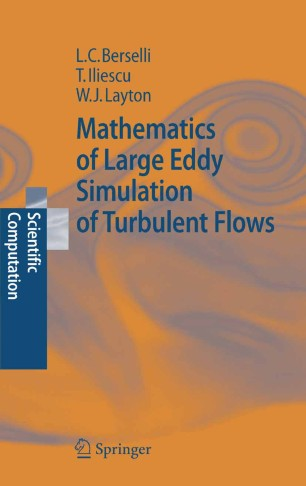# Mathematics of Large Eddy Simulation of Turbulent Flows

• Luigi C. Berselli
• Traian Iliescu
• William J. LaytonBook

Part of the Scientific Computation book series (SCIENTCOMP)

1. Front Matter
Pages I-XVII
2. ### Introduction

1. Pages 3-28
2. Pages 29-68
3. ### Eddy Viscosity Models

1. Pages 71-104
2. Pages 105-122

1. Pages 135-142
2. Pages 143-194
3. Pages 195-224
5. ### Boundary Conditions

1. Pages 227-252
2. Pages 253-272
6. ### Numerical Tests

1. Pages 275-294
2. Pages 295-325
7. Back Matter
Pages 327-350

### Introduction

Large eddy simulation (LES) is a method of scientific computation seeking to predict the dynamics of organized structures in turbulent flows by approximating local, spatial averages of the flow. Since its birth in 1970, LES has undergone an explosive development and has matured into a highly-developed computational technology. It uses the tools of turbulence theory and the experience gained from practical computation.

This book focuses on the mathematical foundations of LES and its models and provides a connection between the powerful tools of applied mathematics, partial differential equations and LES. Thus, it is concerned with fundamental aspects not treated so deeply in the other books in the field, aspects such as well-posedness of the models, their energy balance and the connection to the Leray theory of weak solutions of the Navier-Stokes equations. The authors give a mathematically informed and detailed treatment of an interesting selection of models, focusing on issues connected with understanding and expanding the correctness and universality of LES.

This volume offers a useful entry point into the field for PhD students in applied mathematics, computational mathematics and partial differential equations. Non-mathematicians will appreciate it as a reference that introduces them to current tools and advances in the mathematical theory of LES.

### Keywords

Computational Fluid Dynamics Large Eddy Simulation Navier-Stokes equation Scientific Computing Simulation differential equation modeling partial differential equation

#### Authors and affiliations

• Luigi C. Berselli
• 1
• Traian Iliescu
• 2
• William J. Layton
• 3
1. 1.Department of Applied Mathematics “U. Dini”University of PisaPisaItaly
2. 2.Department of MathematicsVirginia Polytechnic Institute and State UniversityBlacksburgUSA
3. 3.Department of MathematicsUniversity of PittsburghPittsburghUSA